﻿ LDA总结与实例分析

# LDA简介

LDA全称Linear Discriminant Analysis，意为线性判别分析，是一种高效准确的降维方法（只降一维，不精确才难）。简单来说，就是找到一个比原数据集低一维的分类面，让原数据集在此面上投影，投影的结果就是降一维后的新数据集。所以这个面的方向非常重要，它决定了降维后数据的特征保留程度。而我们的LDA求解，就是在研究如何能找到最理想的投影方向。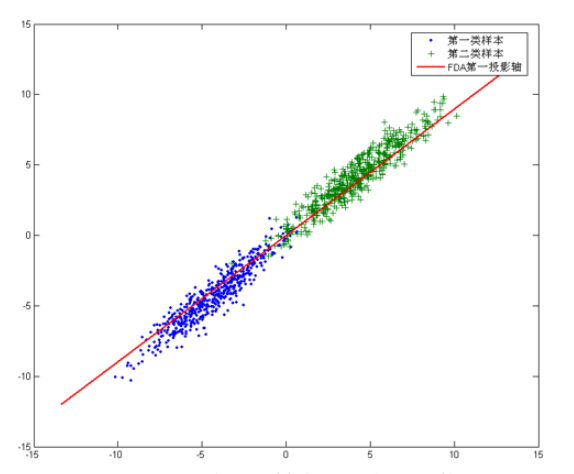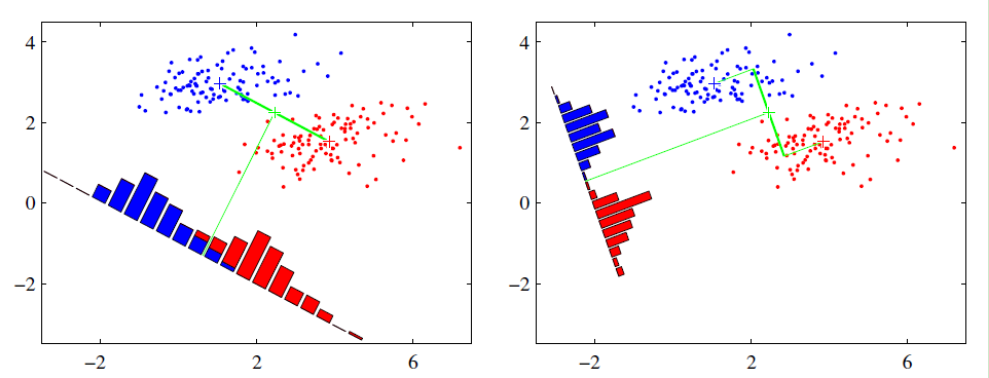# LDA计算过程

## 符号铺垫

w为待求投影面方向， $m_1$$m_2$为第1类第2类数据的均值，也可以说成原始中心：

$m_i = \frac{1}{N_i}\sum\limits_{x \in X_i}x\;\;(i=0,1)$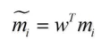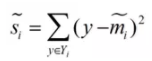## 求解过程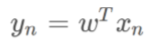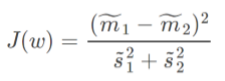$J(w)$可以衡量类内、类外的数据离散程度，使$J(w)$分子最大，分母最小，此时的w就是我们想要的方向。换句话，我们要做的就是最大化$J(w)$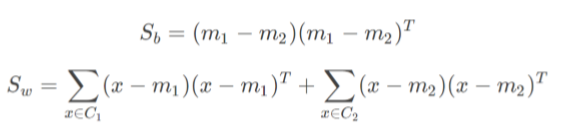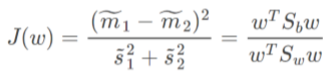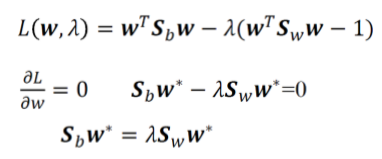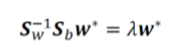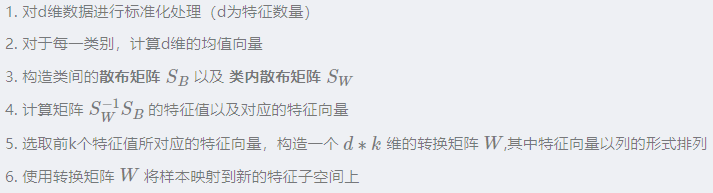# LDA实例分析

• Compute the projection direction using LDA from two sets of points (on the paper by hand)
C1=[(1, 0), (1, 1), (0, 1)]
C2=[(1, 2), (2, 1), (2, 2)]

$S_b$$S_w$可以求解$S_w^{-1}$$S_b$w=$\lambda$w

$S_w^{-1}$$S_b$特征值为：$\lambda_1$=0 $\lambda_2$=3

$\eta_1$=$\begin{pmatrix} 1 \\ -1 \\ \end{pmatrix}$
$\eta_2$=$\begin{pmatrix} 1 \\ 1 \\ \end{pmatrix}$

# LDA优缺点

(1) 计算速度快

(2) 充分利用了先验知识

(1) 当数据不是高斯分布时候，效果不好，PCA也是。

(2) 降维之后的维数最多为类别数-1。

（降维之后的维数最多为类别数-1。所以当数据维度很高，但是类别数少的时候，算法并不适用）